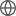Old Web
English
# Rank-One Matrix Approximation with ${\mathcal{l_p}}$-norm for Image Inpainting
In the problem of image inpainting, one popular approach is based on low-rank matrix completion. Compared with other methods which need to convert the image into vectors or dividing the image into patches, matrix completion operates on the whole image directly. Therefore, it can preserve latent information of the two-dimensional image. An efficient method for low-rank matrix completion is to employ the matrix factorization technique. However, conventional low-rank matrix factorization-based methods often require a prespecified rank, which is challenging to determine in practice. The proposed method factorizes an image matrix as a sum of rank-one matrices so that it does not require rank information in advance as it can be automatically estimated by the algorithm itself when the algorithm has satisfactorily converged. In our study, matching pursuit is applied to search for the best rank-one matrix at each iteration. To be robust against impulsive noise, the residual error between the observed and estimated matrices is minimized by $\ell _p$ -norm with $0 . Then the resultant$\ell _p\$ -norm minimization is solved by the iteratively reweighted least squares method. The proposed model is beneficial for the robustness against outliers, and does not require rank information. Experimental results verify the effectiveness and higher accuracy of the proposed method with comparison to several state-of-the-art matrix completion-based image inpainting approaches.